## Odd, Even number checker in Java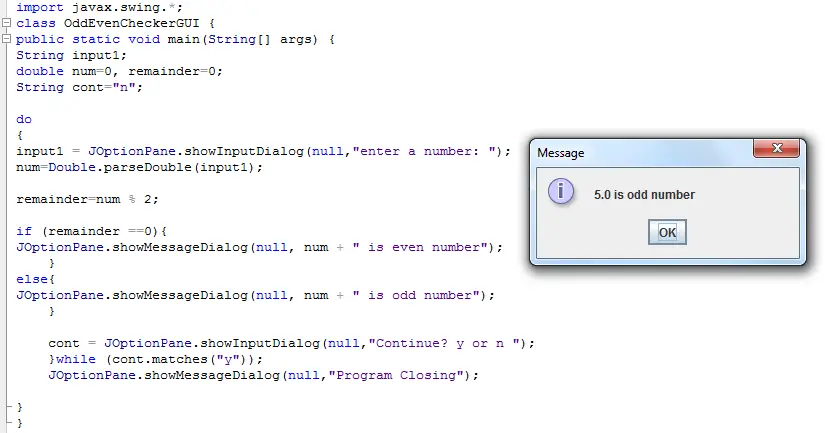Odd, Even number checker in Java

A java program that wil determine if the number entered by the user is an odd or even number. The java program has two versions the gui and the console based program. The gui uses swing library and the console uses scanner class.

Happy programming!

## Basic Math Functions in Java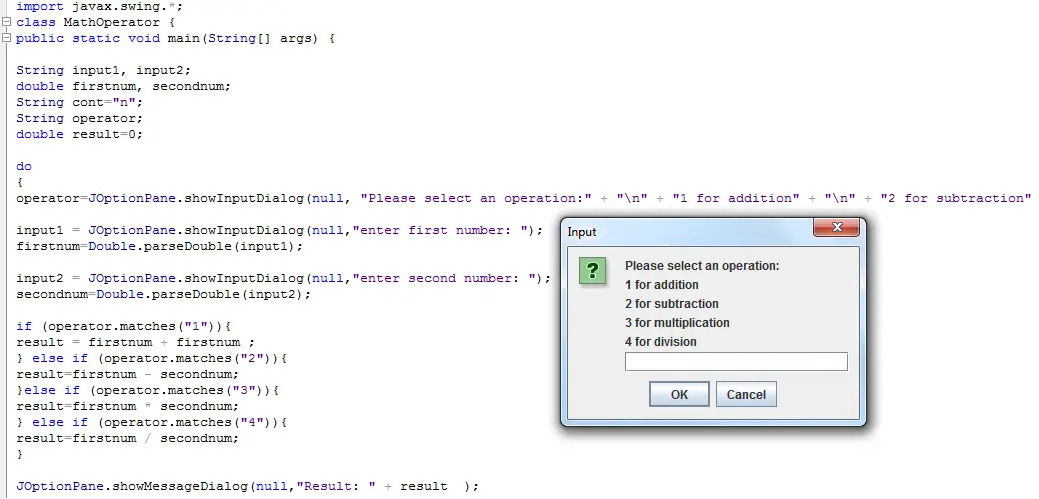Basic Math Functions in Java

This java program is written in console and gui format. The program will demonstrate the basic mathematical operators (add, minus, divide and multiply). It has also a do while loop which allows the user to execute the program again or just close and end it.

## High, Low number checker in Java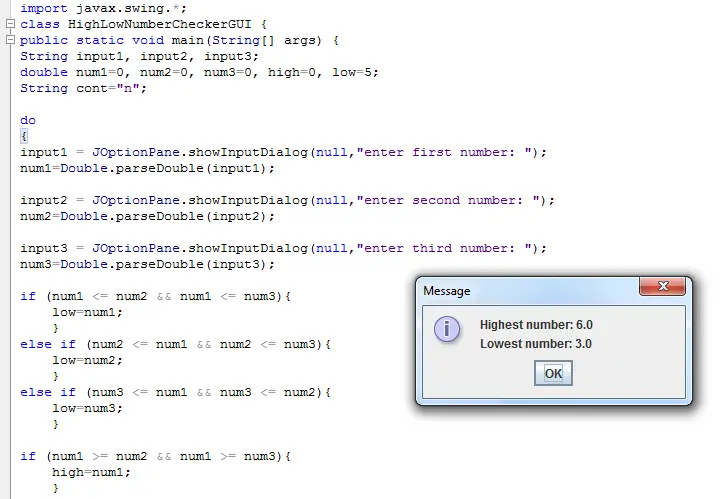High, Low number checker in Java

This sample java program will determine the highest and the lowest number entered by the user. The program will ask the user to enter 3 numbers and the program will display which is the highest and lowest among them. It is also coded in gui and console version.

## Area of Rectangle Solver in Java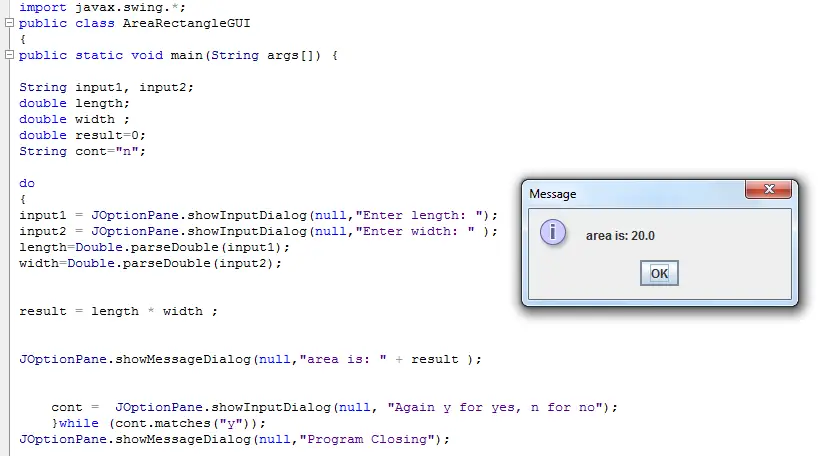Area of Rectangle Solver in Java

It is a very simple program intended for beginners who wants to learn java programming language. The program will computer for the area of a rectangle, the user will be ask to enter the length and the width of the rectangle then the program will display the result. The program is written in gui and console version.

## ComboBox in Java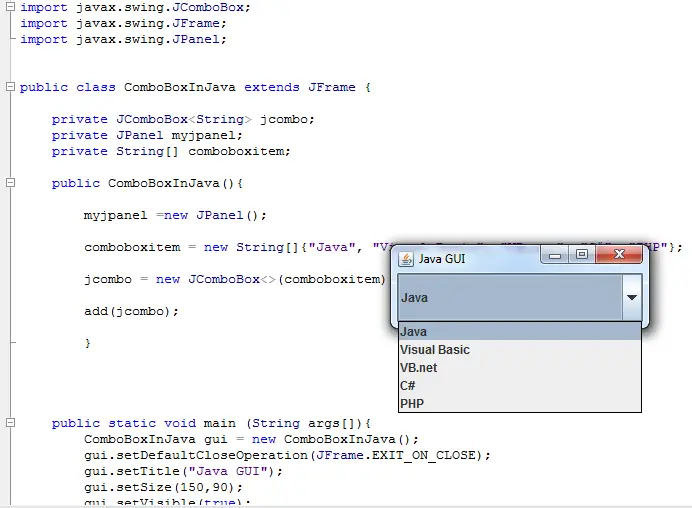ComboBox in Java

A gui program in java that demonstrate how to add a combo box in a window. The program uses swing library. JComboBox is one of the component of swing library.

## Centimeter to Meter converter in JavaCentimeter to Meter converter in Java

This not just a centimeter to meter converter, you can also convert meter to centimeter. This is a java program written in console and gui version that allows the user to select an option whether to convert centimeter to meter or meter to centimeter. This will also allow you to choose whether to close the program or continue and run again the program.

## Celsius to Fahrenheit converter in Java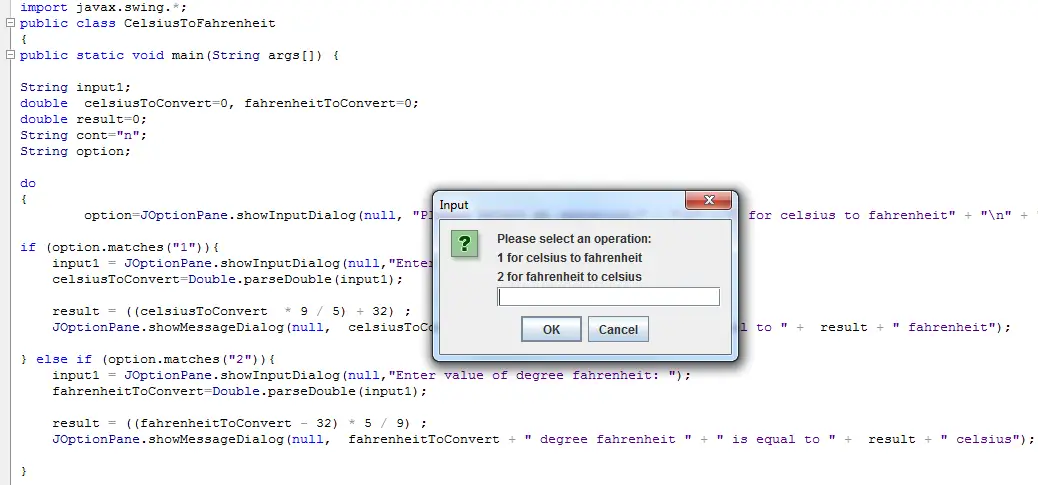Celsius to Fahrenheit converter in Java

Same with the previous program, the user has the option to either convert Celsius to Fahrenheit or Fahrenheit to Celsius.

This is a GUI program in java that uses swing library and JOptionPane message and input dialog for accepting input and displaying the output.

## Total Cost Solver in Java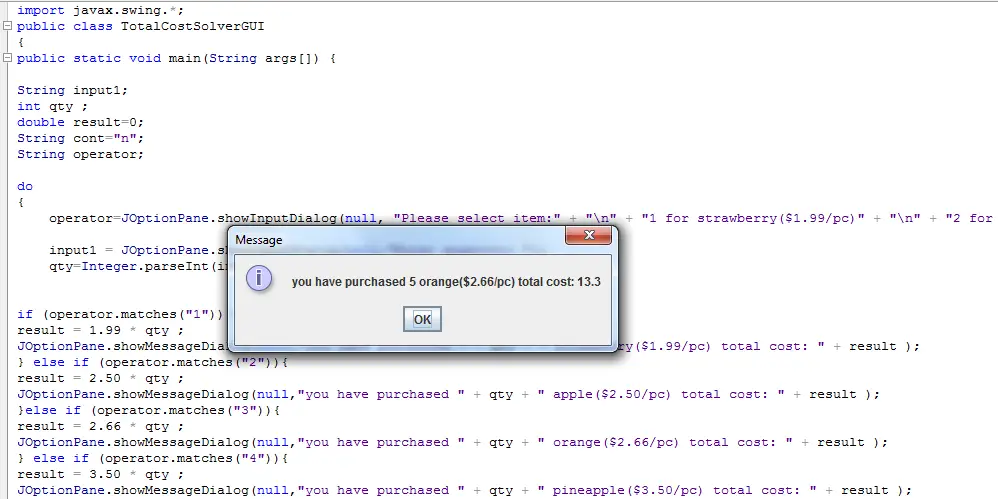Total Cost Solver in Java

A sample source codes in java that will compute the total cost you have purchased. The formula is total cost is equal to the price of the item multiply to the quantity of the item you want to purchase.

Ex:
Apple is \$1.50 each and you want to get 4 apples so the total cost is \$6.
Total cost = \$1.50 x 4 which is \$6.

## Get the Square and Square Root of a number in Java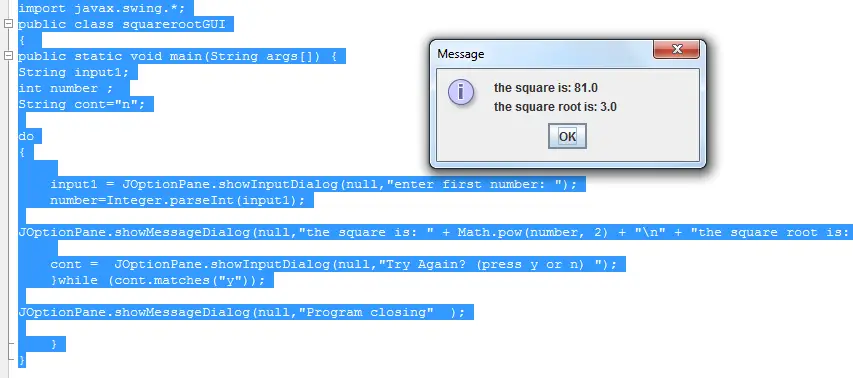Get the Square and Square Root of a number in Java

This java program will accept an input from a user and then the program will display the square and the square root of the number entered by the user.

This is written in console and gui (graphical user interface) version.

## Input Checker in Java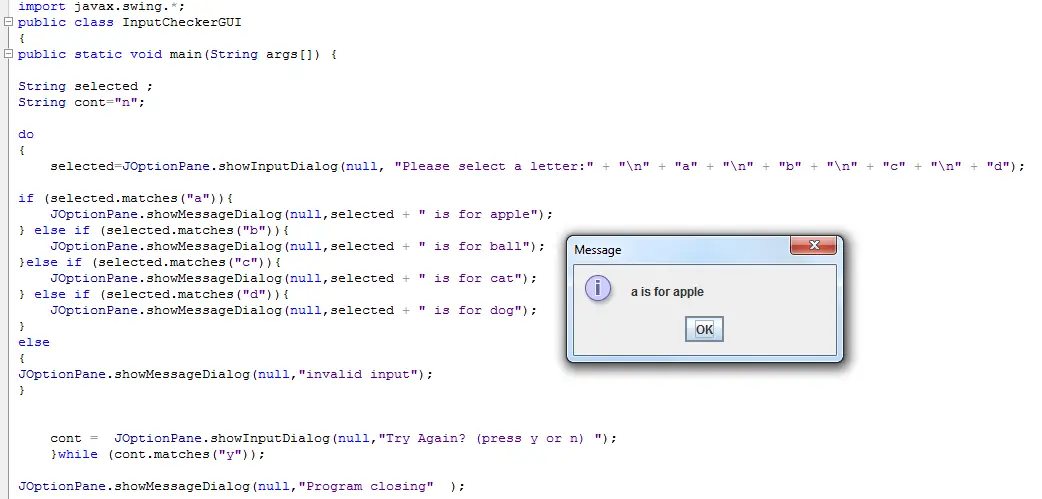Input Checker in Java

It is a java program that will check in the character or string that you have input or entered matches to the criteria set in the program. If a match is found the program will display a message if not a message also displays saying that your input is invalid, which means that it has no match to the given criteria.